# Basic functions - math word problems

#### Number of problems found: 2274

• A farmer's heritage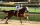A farmer died leaving his 17 horses to his 3 sons. When his sons opened up the Will it read: My eldest son should get 1/2 (half) of total horses; My middle son should be given 1/3rd (one-third) of the total horses; My youngest son should be given 1/9th (o
• Display case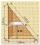Place a glass shelf at the height of 1m from the bottom of the display case in the cabinet. How long platter will we place at this height? The display case is a rectangular triangle with 2 m and 2.5 m legs.
• Similarity n-gon9-gones ABCDEFGHI and A'B'C'D'E'F'G'H'I' are similar. The area of 9-gon ABCDEFGHI is S1=190 dm2 and the diagonal length GD is 32 dm. Calculate area of the 9-gon A'B'C'D'E'F'G'H'I' if G'D' = 13 dm.
• Two machinesPerformances of two machines are in a ratio of 7:12. A machine with less power produced 406 pieces of products per shift. a) How many pieces produced per shift second machine? b) How many pieces produced two machines together for five shifts?
• Birthdate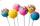Jane on birthday brought 30 lollipops and 24 chewing gum for their friends. How many friends has, if everyone receives the same number of lollipops and chewing gums? How much chewing gum and lollipops got any friend?
• Lookout towerHow high is the lookout tower? If each step was 3 cm lower, there would be 60 more of them on the lookout tower. If it was 3 cm higher again, it would be 40 less than it is now.
• Fewer than 500 sheep,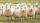There are fewer than 500 sheep, but if they stand in a double, triple, quadruple, five and sixth order, one sheep will remain. But they can stand in the seventh order. How many are sheep?
• Three friendsThree friends divided the profit 104,650 CZK, so that for every 4 CZK, which got the first friend equals 5 crowns for second and for every 9 CZK, which got the second equals 16 CZK for third. Question: Who got the most and how much.
• Three diceThe player throwing the three dice asked G. Galilei the question: "Should I bet on the sum of 11 or the sum of 12?" What did Galilei answer him? Hint: write down all three triples of numbers that can be thrown and: have a total of 11 have a total of 12 an
• Desks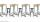A class has 20 students. The classroom consists of 20 desks, with 4 desks in each of 5 different rows. Amy, Bob, Chloe, and David are all friends, and would like to sit in the same row. How many possible seating arrangements are there such that Amy, Bob,
• Shoemaker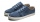Both the shoemaker and his apprentice repaired his shoes. The apprentice worked for 6 days and repaired 10 pairs of shoes every day. The shoemaker did the same job in 4 days. How many pairs of shoes did the shoemaker repair per day?
• Percentages above 100%What is 122% of 185? What is the meaning of percentages above 100%?
• The tentThe tent shape of a regular quadrilateral pyramid has a base edge length a = 2 m and a height v = 1.8 m. How many m2 of cloth we need to make the tent if we have to add 7% of the seams? How many m3 of air will be in the tent?
• The ticketsThe tickets to the show cost some integer number greater than 1. Also, the sum of the price of the children's and adult tickets, as well as their product, was the power of the prime number. Find all possible ticket prices.
• MO-Z5-3-66 tilesThe picture shows a square tiles with side 10 dm which is composed of four identical small rectangles and squares. Circumference of small square is five times smaller than the circumference of the entire tile. Determine the dimensions of the rectangle.
• HallRectangular hall will have pave by square tiles with a side length 15 cm. The hall has length 18 meters and width 3 m. How many tiles need to buy if 2 percent of the amount is disrupted during the work?
• Car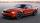The car goes from point A to point B at speed 86 km/h and back 53 km/h. If it goes there and back at speed 67 km/h trip would take 10 minutes shorter. What is the distance between points A and B?
• The florist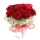A consignment of 200 roses arrived at the florist in the morning. She sold more than half of them during the day. She wants to tie bouquets of the remaining roses. If she ties a bouquet of three, four, five or six roses, there will always be one rose left
• PupilsThere are 32 pupils in the classroom, and girls are two-thirds more than boys. a) How many percents are more girls than boys? Round the result to a whole percentage. b) How many are boys in the class? c) Find the ratio of boys and girls in the class. Writ
• Sugar cubesThe glass has 600 ml of tea, which represents 80% of the volume of the glass. If you put twenty regular sugar cubes of 2 cm in the tea, how many ml of tea are poured?

Do you have an interesting mathematical word problem that you can't solve it? Submit a math problem, and we can try to solve it.

We will send a solution to your e-mail address. Solved examples are also published here. Please enter the e-mail correctly and check whether you don't have a full mailbox.

Please do not submit problems from current active competitions such as Mathematical Olympiad, correspondence seminars etc...